Fluid Mechanics - 1

# Fluid Mechanics - 1

Test Description

## 15 Questions MCQ Test GATE Mechanical (ME) 2023 Mock Test Series | Fluid Mechanics - 1

Fluid Mechanics - 1 for Mechanical Engineering 2023 is part of GATE Mechanical (ME) 2023 Mock Test Series preparation. The Fluid Mechanics - 1 questions and answers have been prepared according to the Mechanical Engineering exam syllabus.The Fluid Mechanics - 1 MCQs are made for Mechanical Engineering 2023 Exam. Find important definitions, questions, notes, meanings, examples, exercises, MCQs and online tests for Fluid Mechanics - 1 below.
Solutions of Fluid Mechanics - 1 questions in English are available as part of our GATE Mechanical (ME) 2023 Mock Test Series for Mechanical Engineering & Fluid Mechanics - 1 solutions in Hindi for GATE Mechanical (ME) 2023 Mock Test Series course. Download more important topics, notes, lectures and mock test series for Mechanical Engineering Exam by signing up for free. Attempt Fluid Mechanics - 1 | 15 questions in 45 minutes | Mock test for Mechanical Engineering preparation | Free important questions MCQ to study GATE Mechanical (ME) 2023 Mock Test Series for Mechanical Engineering Exam | Download free PDF with solutions
 1 Crore+ students have signed up on EduRev. Have you?
Fluid Mechanics - 1 - Question 1

### The relationship between the shear stress (V) and the rate of angular deformation of a fluid is given by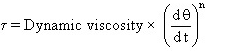The fluid with the exponent n > 1 is known as

Fluid Mechanics - 1 - Question 2

### A Bourdon gauge mounted on a closed pressurized container showing 65 KPa at Hyderabad where the atmospheric pressure is 100 KPa. If the container is now carried to a higher elevation (Ooty) where the atmospheric pressure is 90 KPa, what new reading will be shown by the bourdon gauge at Ooty?

Fluid Mechanics - 1 - Question 3

###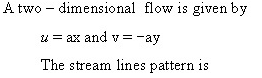Fluid Mechanics - 1 - Question 4

The Bernoulli s equation is written with usual rotation as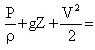constant

In this equation each of the terms represents.

Fluid Mechanics - 1 - Question 5

The highest point of syphon is called as

Fluid Mechanics - 1 - Question 6

If a rectangular plate 4m x 3m is completely submerged under a fluid of relative density 0.8 as
shown in the given figure, then the hydraulic thrust on the plate would be, (Take g= 10m/s2 )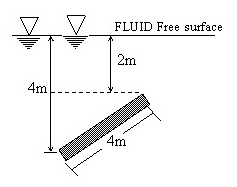Fluid Mechanics - 1 - Question 7

Figure shows a vertical contraction pipe carries water. The water diameter D2 for the condition of P1 = P2 ,in meters (Neglect losses ) is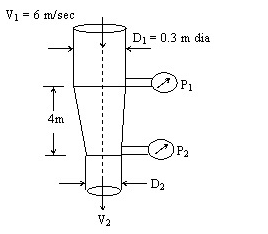Fluid Mechanics - 1 - Question 8

The fluid entering a control volume has specific volume 0.833 m3/kg and a velocity of 5 m/sec, the area of the intake being 20 cm2 . If the fluid leaves the control volume through a 25 mm diameter pipe with a velocity of4 m/sec, the mass flow rate (in kg/sec)
and fluid mass density (in kg/m3 ) respectively are:

Fluid Mechanics - 1 - Question 9

A ram - jet rocket engine consumes 20 kg of air/sec and 0.6 kg of fuel/sec. The exit velocity of the gases is 520 m/sec relative to the engine and the rocket velocity is 200 m/sec absolute. What is the nearly momentum power developed?

*Answer can only contain numeric values
Fluid Mechanics - 1 - Question 10

A pitot - static tube is used to measure air velocity. If a manometer connected to the instrument indicates a difference in pressure head between the tappings of 4 mm of water, calculate the air velocity assuming the coefficient of the pitot tube to be unity. Take density of air = 1.2 kg/mand g = 9.8 m/sec2 .

The velocity of air is  ____  m/sec . ?

*Answer can only contain numeric values
Fluid Mechanics - 1 - Question 11

Water is pumped through a pipe line to a height of 10 m at the rate of 6000 l.p.m, Friction and minor losses are 5m, then the power of pump required is ____ (in KW)
(Take g=10m/s2)

Fluid Mechanics - 1 - Question 12

Match list -I with list -II and choose correct code.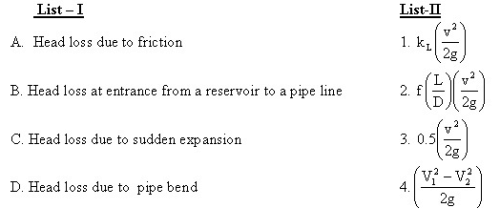Fluid Mechanics - 1 - Question 13

In an automobile traveling at ‘V’ kmph the power expended in over coming resistance is T. If the automobile travels at ‘3 V’ kmph the power expended in over coming wind resistance is,

Fluid Mechanics - 1 - Question 14

Water is flowing through a horizontal pipe of constant diameter and the flow is laminar. If the diameter of the pipe is increased by 50% keeping the volume flowrate constant, them the pressure drop in the pipe due to friction will decrease by

Fluid Mechanics - 1 - Question 15

The head loss in 100 m length of a 0.1 m dia. pipe (f = 0.02) carrying water is 10 m. The boundary shear stress in kPa

## GATE Mechanical (ME) 2023 Mock Test Series

26 docs|239 tests
 Use Code STAYHOME200 and get INR 200 additional OFF Use Coupon Code
Information about Fluid Mechanics - 1 Page
In this test you can find the Exam questions for Fluid Mechanics - 1 solved & explained in the simplest way possible. Besides giving Questions and answers for Fluid Mechanics - 1, EduRev gives you an ample number of Online tests for practice

## GATE Mechanical (ME) 2023 Mock Test Series

26 docs|239 tests